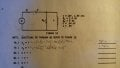# RC-RL series parallel question

#### LymanT

Joined Sep 25, 2014
24
Hey buds,

Doing a review packet to refresh some forgotten methods for future opportunities and I have come across another question that I am stuck on. I've searched online but can't find a clear approach to this problem. This is probably simple for you guys but its been years since I've been submerged in circuit analysis. It feels good to relearn the things I've forgotten.

For this RC-RL series parallel circuit, I can calculate the reactance for the the inductor and cap, but how to calculate the total impedance is what I can't figure out. I can get the impedance for the RL parallel, but how to get total impedance for the circuit is where I'm stuck. Calculating the phi is another unknown for me. Any tips, advice, info, will be helpful.

Thanks guys#### WBahn

Joined Mar 31, 2012
29,494
You need to either show enough work for us to see what techniques you know, or let us know what approaches you have available to you (which, admittedly, is hard for someone to know that they need to do that since they often only know one approach and aren't even aware that other approaches exist).

When working with impedances, the best way (I believe) is using complex impedance. But that requires that you be conversant with complex numbers and arithmetic. Are you? If not, then you probably have a slew of formulas that tell you how to combine things using magnitudes and angles for a bunch of different situations. Does that sound familiar?

#### LymanT

Joined Sep 25, 2014
24
For the RC series, I calculate an impedance of 3535ohms.
For the RL parallel, I calculate the same impedance of 3535ohms.

The methods that I have been using to analyze AC series and parallel have not been complex. My old textbook covers these topics in complex as well. Being unfamiliar or rather have forgotten, I've started learning basic complex numbers again. I have not gotten to where complex numbers are applied to AC circuits. I had just learned how to calculate admittance, conductance, and susceptance for AC parallel, so thats where I stand on working my way thru.

arctan (X/R) = angle for series
arctan (B/G) = angle for parallel

The part that confuses me too, is the Siemens within parallel. Voltage is common in a parallel circuit. It seems I just use the siemens for finding the angle...

#### LymanT

Joined Sep 25, 2014
24
After further reading my textbook, I come to find that the problems are solved using rectangular form and polar form. It's been awhile since I've punched away at my TI89...

#### MrAl

Joined Jun 17, 2014
10,898
Hi,

I havent used my TI89 in a long time either. Long time back i used the TI85 for lots of stuff, daily, and ran the batteries down almost every dayIf you get back into complex arithmetic you will be able to solve these problems fast. The only difference really is the way you add, subtract, multiply, and divide complex numbers. Maybe a few other small rules and you get going pretty fast.

In the complex number world, the inductor impedance is jwL and the cap 1/(jwC) and from there it is just a matter of using what you know about combining resistors to solve for the total impedance. It is well worth looking into because it applies to so many circuits. There are also transforms that take you from the frequency domain (AC really) to the time domain, so you get the time response if you need it. This all starts from the complex number view of things.

If you are interested i could show you a set of rules that would take no more than a few lines that would cover a large number of problems.

#### LymanT

Joined Sep 25, 2014
24
Hi,

I havent used my TI89 in a long time either. Long time back i used the TI85 for lots of stuff, daily, and ran the batteries down almost every dayIf you get back into complex arithmetic you will be able to solve these problems fast. The only difference really is the way you add, subtract, multiply, and divide complex numbers. Maybe a few other small rules and you get going pretty fast.

In the complex number world, the inductor impedance is jwL and the cap 1/(jwC) and from there it is just a matter of using what you know about combining resistors to solve for the total impedance. It is well worth looking into because it applies to so many circuits. There are also transforms that take you from the frequency domain (AC really) to the time domain, so you get the time response if you need it. This all starts from the complex number view of things.

If you are interested i could show you a set of rules that would take no more than a few lines that would cover a large number of problems.

Thanks MyAl.

Yeah, the complex numbers are long forgotten but I've been brushing up on it. It feels good to relearn these and I know it will come in handy later. Sure, any help will be appreciated. I'm actually working my way thru kahnacademy's library of online learning sessions. I'm actually learning basic stuff I don't know if I ever learned in the first place. Not necessarily in an undergraduate program but things as basic as algebra and trig. I never quite understood certain things but that academy is breaking it down to where I am catching on easily.

After reviewing my textbook further I was able to understand the way they were analyzing this type of circuit with rectangular and polar forms. What I ended up with was:

Zt = 1/Y1 + R + (-j)Xc.
Zt = 5000ohms<0 degrees

Learning about G, Y, and B in AC parallel was something I don't remember. So it took some time to understand it.